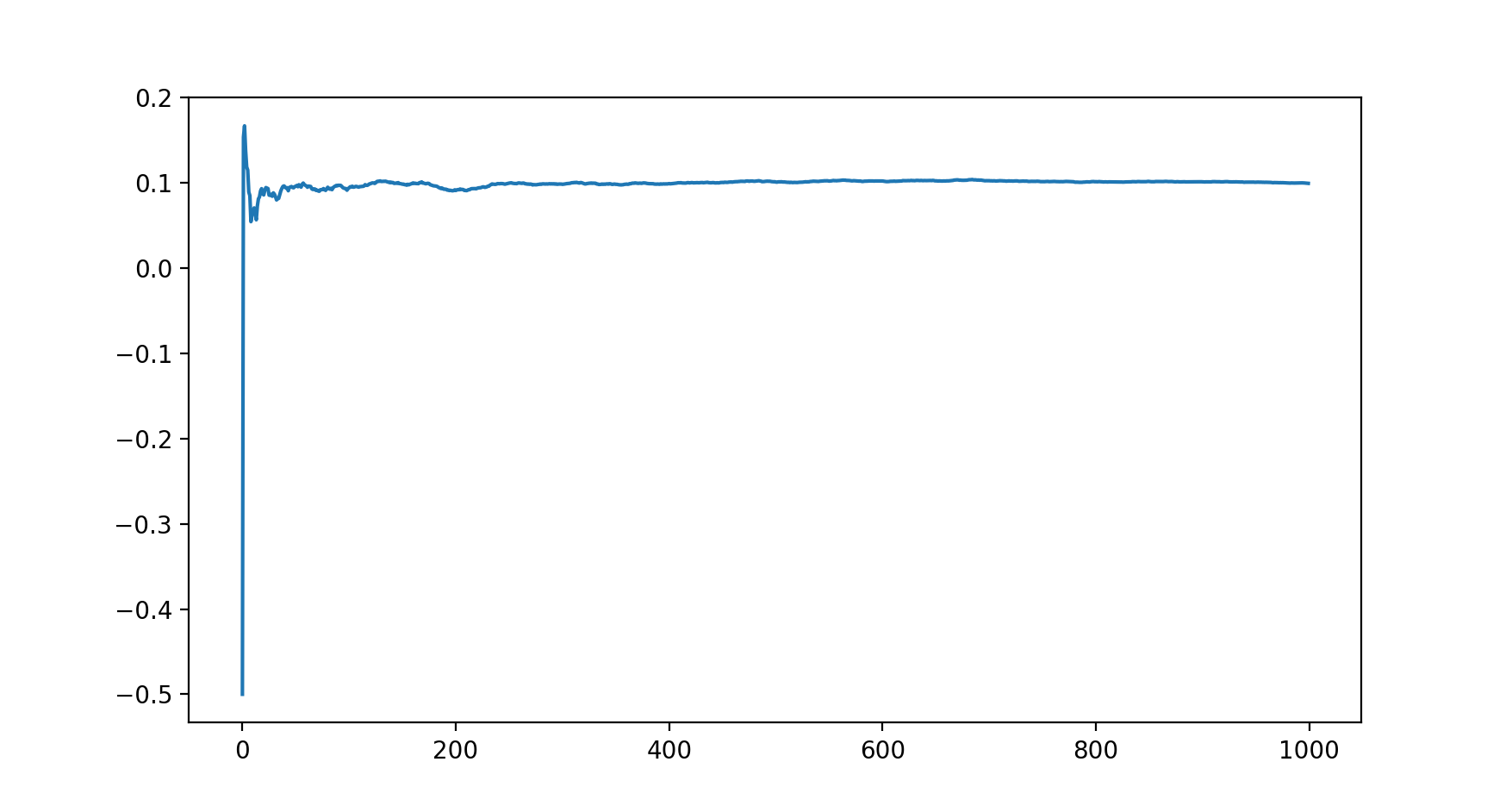In :
%matplotlib notebook
import collections

import numpy as np
import numpy.random as rnd

import matplotlib.pyplot as plt

rnd.seed(0)

In :
REAL_DISEASE_FREQ = 0.1

N_SAMPLES = 100000

In :
def simulate_single_answer():
# Honest answer: True if has disease, False otherwise
if rnd.random() < 0.5:
return rnd.random() < REAL_DISEASE_FREQ
# Fake answer. Random choice with probability .5
else:
return rnd.random() < 0.5

In :
database = [simulate_single_answer() for i in range(N_SAMPLES)]


## Frequentist estimation of p¶

=> p = 2*(#True/#Total - 0.25)

In :
def estimate_p(db):
counter = collections.Counter(db)
return 2*(counter[True]/len(db) - 0.25)

print('Estimated p:', estimate_p(database))

Estimated p: 0.09919999999999995


## Convergence plot¶

In :
estimations = [estimate_p(database[:i]) for i in range(1, N_SAMPLES, 100)]

plt.plot(estimations)Out:
[<matplotlib.lines.Line2D at 0x105e8c828>]